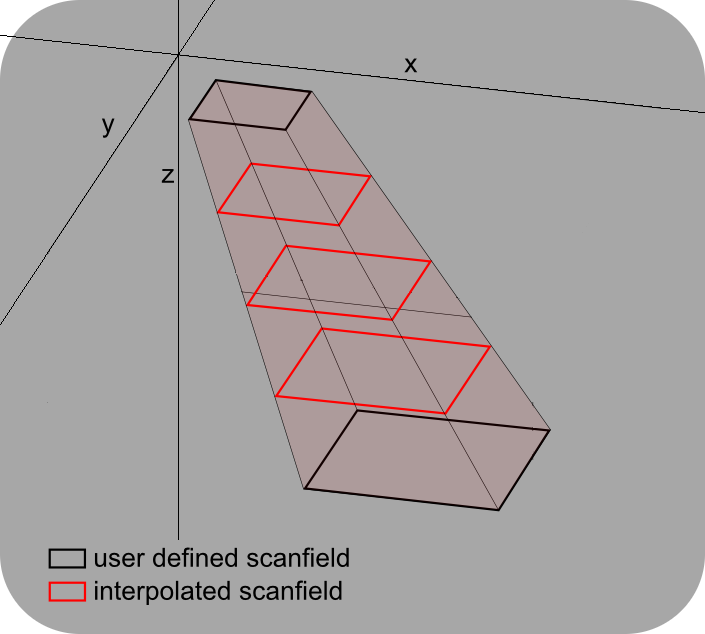# ScanImage® Coordinate Systems¶

Each ScanImage® scanner (ResScan / LinScan) uses its own normalized coordinate system to allow for different field of views. The scanner coordinates are mapped into a common reference space. The reference space is used in the ScanImage® API and all GUIs exposed to the user.

## Scanner Coordinates¶

The scanner coordinates are a normalized coordinate system with x and y extending from 0 to 1. This extent is regardless of the size and the aspect ratio of the actual scanner field of view and defines the maximum addressable space of the scanner.

 Left: Zoom 1x, spatial fillfraction 0.7 Right: Zoom 2x, spatial fillfraction 0.7

## Reference Coordinates¶

The scanner spaces are mapped into a common reference space via affine transformations. All Scanfields and stimuli are defined in reference space and then mapped back into the appropriate scanner space to control the scanner hardware. The aspect ratios in reference space show the true proportions of the scanners in the system.

To simplify the alignment of the scanners relative to each other, ScanImage® features an Alignment Controls Window. After a successful alignment, ScanImage® stores the affine matrices used for the transformation as properties in the imaging system objects:

```hSI.hResScan.scannerToRefTransform % affine transformation for ResScan
hSI.hLinScan.scannerToRefTransform % affine transformation for LinScan
```

Attention

The alignment controls window is only available in Premium ScanImage.

Note

Currently ScanImage® only supports translation and scaling. Support for rotation will be added at a later point. When editing the transformations manually, adding a rotational component will yield unpredictable results.Reference Space¶ Example for mapping Scanner coordinates to Reference Space ResScan: Note that the angular range of the y-galvo is twice the angular range of the resonant scanner in this example. The aspect ratio in Reference Space displays the proportions correctly. The scanfield in ResScan space appears distorted because of the normalized scanner coordinates, but simplifies the algorithms used for hardware control.

## Scanfields, ROIs, ROI Groups¶

### Scanfields¶

A scanfield is the 2D cross section of a ROI at a particular z plane. The discrete set of user defined scanfields defines the volumetric shape of the ROI.

Each scanfield is defined by the following set of properties:

• rectangle: x,y coordinates of the top left corner; width; height

• scan resolution: pixels per line, number of lines

• rotation angle

All coordinates are defined in a normalized full field of view coordinate system, which extends from 0 to 1 in both x and y direction.Scanfields¶ Scanfields are defined in a normalized full field of view coordinate system, which is defined by the maximum deflection of the galvanometric mirrors.

### ROIs¶

A ROI is a three dimensional volume defined by a set of one or more scanfields, each at a unique z-level. A ROI which contains only one scanfield stretches from z = -inf..inf. To limit the z extent of the ROI, define at least two second scanfields. Each scanfield is a control point in the shape of the 3D volume. Between control points, scanfields are interpolated. Alternatively, a ROI can exist only at discrete z planes only where scanfields are defined.

Note

The scanfields that you add to a ROI do not specify the z planes that will actually be imaged when you start an acquisition. The scanfields are only defining the shape of the 3D volume. The z planes to be imaged are set up through the FastZ GUI. For each z plane to be imaged, ScanImage® will look at the current ROI group to determine what ROIs exist at that z plane and extract the scanfield to be imaged.

Ex: In the example ROI on the left, scanfields are are defined at z planes of 0 and 10. For this example, suppose the user sets up a fast Z for 4 slices, 4 steps (microns) per slice. The actual z planes that will be imaged would be 0, 4, 8, and 12. The user defined scanfield 1 would be imaged at z plane 0, while interpolated scanfields between 0 and 10 would be imaged at z of 4 and 8. Nothing would be imaged at z of 12 because the ROI only exists between the top and bottom scanfields.ROIs¶ x y width height z scanfield 1 0.1 0.1 0.2 0.2 0 scanfield 2 0.5 0.5 0.4 0.4 10 A ROI consisting of two user defined scanfields at z=0 and z=10. If needed, scanfields are linearly interpolated for different values of z (red rectangles).

### ROI Group¶

A collection of ROIs forms a ROI Group. During a volume acquisition, ScanImage® queries the ROI group to return the scanfields at a particular z plane. ScanImage® acquires images for each of the scanfields before moving on to the next z plane.